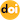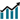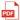# MODIFIKASI METODE RUNGE-KUTTA ORDE EMPAT KLASIK MENGGUNAKAN KOMBINASI DERET LEHMER DENGAN P = 1 DAN P = 4

• Fadilla Ulfa Program Studi Matematika, Fakultas Sains dan Teknologi, UIN Sultan Syarif Kasim Riau
• - Wartono Program Studi Matematika, Fakultas Sains dan Teknologi, UIN Sultan Syarif Kasim Riauhttps://doi.org/10.37859/sainstekes.v1i0.1582

### Abstract

Fourth order classical Runge Kutta method (RK4) is one of numerical method used to solve first order differential equation. In this research describe about modification of RK4 method using combination of Lehmer means with p = 1 and p = 4. Modification of fourth order Runge Kutta method using combination of Lehmer means (RKKCCL) obtainted by replacing aritmatic means with convex combination Lehmer means for p = 1 and p = 4. Based on the result study, the truncation error and stability value of RKKCCL were obtained at the fifth order. Numerical simulation result shows that the error of RKKCCL method is better then RK4 method for y' y, y' y at   0,2 while for y' = 1/y, the error of RKKCCL is almost the same as RK4 method.Abstract views: 21 ,pdf (Bahasa Indonesia) downloads: 96